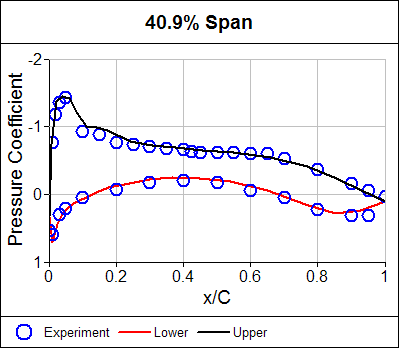# Pressure Coefficient

The pressure coefficient is a dimensionless quantity of interest to aerodynamicists.

The pressure coefficient is defined as:

p - pinf = qinf . Cp

where:
Cp is the dimensionless pressure coefficient
p is the pressure due to the movement of the body through the fluid
pinf is the free stream fluid pressure
p - pinf is known as the gauge pressure
qinf is the free stream fluid dynamic pressure from Bernoulli's equation:

qinf = 1/2 rhoinf . Vinf2

where:
rhoinf is the free stream fluid density
Vinf is the free stream fluid speedPressure Coefficient Comparison Between Calculation (lines) and Experiment Around an Airfoil

You will often see pressure coefficient plots of numerical calculations compared with wind tunnel experimental data as a means to judge the accuracy of the numerical technique used. This type of comparison is often referred to as a validation.